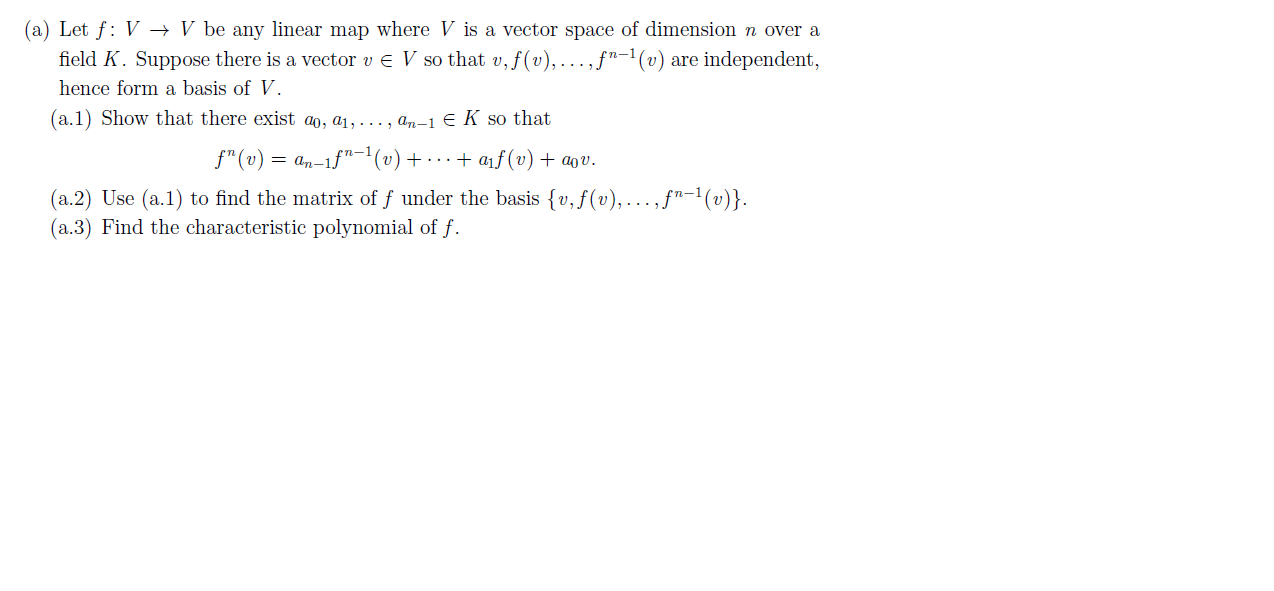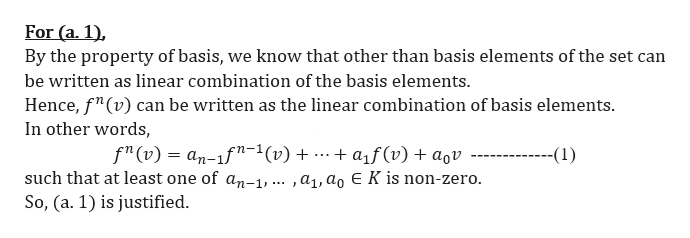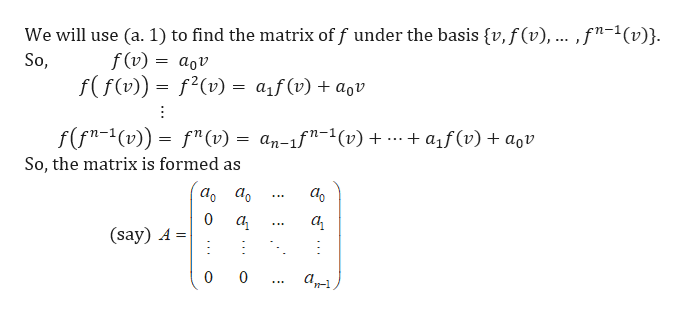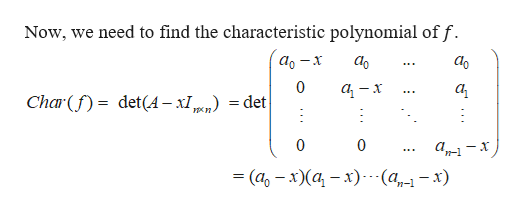# (a) Let f: V > V be any linear map where V is a vector space of dimension n over afield K. Suppose there is a vector v E V so that v, f(v),... ,f"-1 (v) are independent,hence form a basis of V.(a.1 Show that there exist a, ai, ..., an-1 E K so thatf"(v)= an-1f"(v) + ... + af(v) + a0v.(a.2) Use (a.1) to find the matrix of f under the basis {v,f(v),... ,f"-1 (v)}(a.3) Find the characteristic polynomial of f

Question
93 viewshelp_outlineImage Transcriptionclose(a) Let f: V > V be any linear map where V is a vector space of dimension n over a field K. Suppose there is a vector v E V so that v, f(v),... ,f"-1 (v) are independent, hence form a basis of V. (a.1 Show that there exist a, ai, ..., an-1 E K so that f"(v)= an-1f"(v) + ... + af(v) + a0v. (a.2) Use (a.1) to find the matrix of f under the basis {v,f(v),... ,f"-1 (v)} (a.3) Find the characteristic polynomial of f fullscreen
check_circle

Step 1

Let f : V -->V be any linear map where V is a vector space of dimension n over a field K. Suppose there is a vector v ∈ V so that v, f(v), … ,fn-1(v) are independent, hence for a basis.help_outlineImage TranscriptioncloseFor (a. 1) By the property of basis, we know that other than basis elements of the set can be written as linear combination of the basis elements Hence, f"(v) can be written as the linear combination of basis elements. In other words f"(v) an-1f"-1(v) + .-. + af(v) + aqv ---- such that at least one of an-1, ... ,a1,ag E K is non-zero --(1) So, (a. 1) is justified. fullscreen
Step 2

For (a. 2),help_outlineImage TranscriptioncloseWe will use (a. 1) to find the matrix of f under the basis {v, f(v), .. ,f"-1(v)}. f(v) So, aov f(f(v))= f2(v) = aif (v) + agv f(fn-1(v)) fn (v) = an-1f"-1(v) a1f(v)aov So, the matrix is formed as аo а. а 0 а а (say) A 0 0 fullscreen
Step 3

For (a. 3),

...help_outlineImage TranscriptioncloseNow, we need to find the characteristic polynomial of f. аo аo 0 а, Char( det(A-x1 =det 0 0 3 (а, — х)(а - х) -(а, — х) fullscreen

### Want to see the full answer?

See Solution

#### Want to see this answer and more?

Solutions are written by subject experts who are available 24/7. Questions are typically answered within 1 hour.*

See Solution
*Response times may vary by subject and question.
Tagged in

### Math